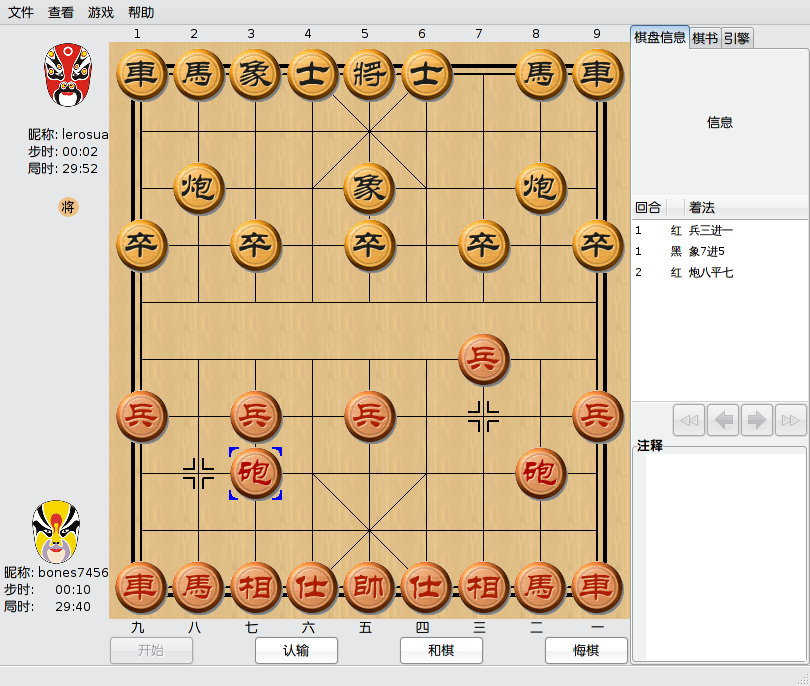# 为什么python里要 if __name__ == ‘__main__’:

```def main(): #具体代码   if __name__ == '__main__': main()```

`Exception AttributeError: "'NoneType' object has no attribute 'population'" in > ignored`

# python中的socket代理

```proxy_handler = urllib2.ProxyHandler({'http' : 'http://地址:端口'}) opener = urllib2.build_opener(proxy_handler, urllib2.HTTPHandler) urllib2.install_opener(opener)```

```import socks, socket socks.setdefaultproxy(socks.PROXY_TYPE_SOCKS5, "地址", 端口) socket.socket = socks.socksocket```

# 跨平台共享鼠标键盘

synergy-plus是一个跨平台的鼠标键盘共享软件，支持linux、mac、windows，可以用一套鼠标键盘控制多台电脑，实现光标从这个屏幕飞到那个屏幕，哈哈。`./synergyc -f t-liushun`

```section: screens LLY: switchCorners = none switchCornerSize = 0 t-liushun: switchCorners = none switchCornerSize = 0 end section: links LLY: right = t-liushun t-liushun: left = LLY end section: options end```

`./synergys -f --config 配置文件名`

========update========

# 没事就和朋友下下棋，pidgin象棋插件偷跑版

gmchess是一款由阳光灿烂的lerosua写的linux下开源的中国象棋程序，使用gtkmm和C++完成。支持人机对战和人人对战。但是之前只能在同一台机子上进行人人对战，这显然很不方便。# 一个支持上传的简单http server

`python -m SimpleHTTPServer`# python的正则表达式 re

```>>> import re >>> s = '\x5c' #0x5c就是反斜杆 >>> print s \ >>> re.match('\\\\', s) #这样可以匹配 <_sre.SRE_Match object at 0xb6949e20> >>> re.match(r'\\', s) #这样也可以 <_sre.SRE_Match object at 0x80ce2c0> >>> re.match('\\', s) #但是这样不行 Traceback (most recent call last): File "<stdin>", line 1, in <module> File "/usr/lib/python2.6/re.py", line 137, in match return _compile(pattern, flags).match(string) File "/usr/lib/python2.6/re.py", line 245, in _compile raise error, v # invalid expression sre_constants.error: bogus escape (end of line) >>>```

`'.'`

`'^'`

`'\$'`

```>>> re.findall('(foo.\$)', 'foo1\nfoo2\n') ['foo2'] >>> re.findall('(foo.\$)', 'foo1\nfoo2\n', re.MULTILINE) ['foo1', 'foo2'] >>> re.findall('(\$)', 'foo\n') ['', '']```

`'*'`

`'+'`

`'?'`

`*?``+?``??`

```>>> re.findall('<(.*)>', '<H1>title</H1>') ['H1>title</H1'] >>> re.findall('<(.*?)>', '<H1>title</H1>') ['H1', '/H1']```

`{m}`
m是一个数字，指定将前面的RE重复m次。

`{m,n}`
m和n都是数字，指定将前面的RE重复m到n次，例如`a{3,5}`匹配3到5个连续的a。注意，如果省略m，将匹配0到n个前面的RE；如果省略n，将匹配n到无穷多个前面的RE；当然中间的逗号是不能省略的，不然就变成前面那种形式了。

`{m,n}?`

`'\'`

`[]`

`'|'`

`(...)`

`(?...)`

`(?iLmsux)`
`'i'``'L'``'m'``'s'``'u'``'x'`里的一个或多个字母。表达式不匹配任何字符，但是指定相应的标志：re.I(忽略大小写)、re.L(依赖locale)、re.M(多行模式)、re.S(.匹配所有字符)、re.U(依赖Unicode)、re.X(详细模式)。关于各个模式的区别，下面会有专门的一节来介绍的。使用这个语法可以代替在`re.compile()`的时候或者调用的时候指定flag参数。

```>>> re.findall('(?m)(foo.\$)', 'foo1\nfoo2\n') ['foo1', 'foo2']```

`(?:...)`

`(?P<name>...)`

```>>> m=re.match('(?P<var>[a-zA-Z_]\w*)', 'abc=123') >>> m.group('var') 'abc' >>> m.group(1) 'abc'```

`(?P=name)`

```>>> re.match('<(?P<tagname>\w*)>.*</(?P=tagname)>', '<h1>xxx</h2>') #这个不匹配 >>> re.match('<(?P<tagname>\w*)>.*</(?P=tagname)>', '<h1>xxx</h1>') #这个匹配 <_sre.SRE_Match object at 0xb69588e0>```

`(?#...)`

`(?=...)`

`(?!...)`

`(?<=...)`

```>>> m = re.search('(?<=-)\w+', 'spam-egg') >>> m.group(0) 'egg'```

`(?<!...)`

`(?(id/name)yes-pattern|no-pattern)`

`\number`

`\A`

`\b`

`\B`
`\b`相反，`\B`匹配非单词边界。也依赖于`UNICODE``LOCALE`这两个标志位。

`\d`

```#\u2076\和u2084分别是上标的6和下标的4，属于unicode的DIGIT >>> unistr = u'\u2076\u2084abc' >>> print unistr ⁶₄abc >>> print re.findall('\d+', unistr, re.U) ⁶₄```

`\D`
`\d`相反，不多说了。

`\s`

`\S`
`\s`相反，也不多说。

`\w`

`\W`
`\w`相反，不多说。

`\Z`

python提供了两种基于正则表达式的操作：匹配（match）从字符串的开始检查字符串是否个正则匹配。而搜索（search）检查字符串任意位置是否有匹配的子串（perl默认就是如此）。

```>>> re.match("c", "abcdef") # 不匹配 >>> re.search("c", "abcdef") # 匹配 <_sre.SRE_Match object at ...>```

`re`.compile(pattern[, flags])

```prog = re.compile(pattern) result = prog.match(string)```
`result = re.match(pattern, string)`

```>>> timeit.timeit( ... setup='''import re; reg = re.compile('<(?P<tagname>\w*)>.*</(?P=tagname)>')''', ... stmt='''reg.match('<h1>xxx</h1>')''', ... number=1000000) 1.2062149047851562 >>> timeit.timeit( ... setup='''import re''', ... stmt='''re.match('<(?P<tagname>\w*)>.*</(?P=tagname)>', '<h1>xxx</h1>')''', ... number=1000000) 4.4380838871002197```

`re`.I
`re`.IGNORECASE

`re`.L
`re`.LOCALE
`\w``\W``\b``\B``\s``\S`依赖当前的locale。

`re`.M
`re`.MULTILINE

`re`.S
`re`.DOTALL

`re`.U
`re`.UNICODE
`\w``\W``\b``\B``\d``\D``\s``\S`依赖Unicode库。

`re`.X
`re`.VERBOSE

```a = re.compile(r"""\d + # the integral part \. # the decimal point \d * # some fractional digits""", re.X) b = re.compile(r"\d+\.\d*")```

`re`.search(pattern, string[, flags])

`re`.match(pattern, string[, flags])

`re`.split(pattern, string[, maxsplit=0])

```>>> re.split('\W+', 'Words, words, words.') ['Words', 'words', 'words', ''] >>> re.split('(\W+)', 'Words, words, words.') ['Words', ', ', 'words', ', ', 'words', '.', ''] >>> re.split('\W+', 'Words, words, words.', 1) ['Words', 'words, words.']```

```>>> re.split('(\W+)', '...words, words...') ['', '...', 'words', ', ', 'words', '...', '']```

```>>> re.split('x*', 'foo') ['foo'] >>> re.split("(?m)^\$", "foo\n\nbar\n") ['foo\n\nbar\n']```

`re`.findall(pattern, string[, flags])

```#简单的findall >>> re.findall('\w+', 'hello, world!') ['hello', 'world'] #这个返回的就是元组的列表 >>> re.findall('(\d+)\.(\d+)\.(\d+)\.(\d+)', 'My IP is 192.168.0.2, and your is 192.168.0.3.') [('192', '168', '0', '2'), ('192', '168', '0', '3')]```

`re`. finditer(pattern, string[, flags])

>>> for m in re.finditer('\w+', 'hello, world!'):
... print m.group()
...
hello
world

`re`.sub(pattern, repl, string[, count])

```>>> re.sub(r'def\s+([a-zA-Z_][a-zA-Z_0-9]*)\s*\(\s*\):', ... r'static PyObject*\npy_\1(void)\n{', ... 'def myfunc():') 'static PyObject*\npy_myfunc(void)\n{'```

```>>> def dashrepl(matchobj): ... if matchobj.group(0) == '-': return ' ' ... else: return '-' >>> re.sub('-{1,2}', dashrepl, 'pro----gram-files') 'pro--gram files'```

```>>> re.sub('x*', '-', 'abcxxd') '-a-b-c-d-'```

```>>> re.sub('-(\d+)-', '-\g<1>0\g<0>', 'a-11-b-22-c') 'a-110-11-b-220-22-c'```

`re`.subn(pattern, repl, string[, count])

，还是用例子说话：

```>>> re.subn('-(\d+)-', '-\g<1>0\g<0>', 'a-11-b-22-c') ('a-110-11-b-220-22-c', 2)```

`re`.escape(string)
string中，除了字母和数字以外的字符，都加上反斜杆。

```>>> print re.escape('abc123_@#\$') abc123\_\@\#\\$```

exception `re`.error

match(string[, pos[, endpos]])

pos是开始搜索的位置，默认为0。endpos是搜索的结束位置，如果endpospos还小的话，结果肯定是空的。也就是说只有posendpos-1 位置的字符串将会被搜索。

```>>> pattern = re.compile("o") >>> pattern.match("dog") # 开始位置不是o，所以不匹配 >>> pattern.match("dog", 1) # 第二个字符是o，所以匹配 <_sre.SRE_Match object at ...>```

search(string[, pos[, endpos]])

split(string[, maxsplit=0])
findall(string[, pos[, endpos]])
finditer(string[, pos[, endpos]])
sub(repl, string[, count=0])
subn(repl, string[, count=0])

flags

```>>> pattern = re.compile("o", re.S|re.U) >>> pattern.flags 48```

groups
RE所含有的组的个数。

groupindex

```这个正则有3个组，如果匹配到，第一个叫区号，最后一个叫分机号，中间的那个未命名 >>> pattern = re.compile("(?P<quhao>\d+)-(\d+)-(?P<fenjihao>\d+)") >>> pattern.groups 3 >>> pattern.groupindex {'fenjihao': 3, 'quhao': 1}```

pattern

```>>> print pattern.pattern (?P<quhao>\d+)-(\d+)-(?P<fenjihao>\d+)```

Match对象

`re`.MatchObject被用于布尔判断的时候，始终返回True，所以你用 if 语句来判断某个 match() 是否成功是安全的。

expand(template)
template做为模板，将MatchObject展开，就像sub()里的行为一样，看例子：

```>>> m = re.match('a=(\d+)', 'a=100') >>> m.expand('above a is \g<1>') 'above a is 100' >>> m.expand(r'above a is \1') 'above a is 100'```

group([group1, ...])

```>>> m = re.match(r"(\w+) (\w+)", "Isaac Newton, physicist") >>> m.group(0) # 整个匹配 'Isaac Newton' >>> m.group(1) # 第一个子串 'Isaac' >>> m.group(2) # 第二个子串 'Newton' >>> m.group(1, 2) # 多个子串组成的元组 ('Isaac', 'Newton')```

```>>> m = re.match(r"(?P<first_name>\w+) (?P<last_name>\w+)", "Malcolm Reynolds") >>> m.group('first_name') 'Malcolm' >>> m.group('last_name') 'Reynolds'```

```>>> m = re.match(r"(..)+", "a1b2c3") # 匹配到3次 >>> m.group(1) # 返回的是最后一次 'c3'```

groups([default])

```>>> m = re.match(r"(\d+)\.(\d+)", "24.1632") >>> m.groups() ('24', '1632')```

default的作用：

```>>> m = re.match(r"(\d+)\.?(\d+)?", "24") >>> m.groups() # 第二个默认是None ('24', None) >>> m.groups('0') # 现在默认是0了 ('24', '0')```

groupdict([default])

```>>> m = re.match(r"(?P<first_name>\w+) (?P<last_name>\w+)", "Malcolm Reynolds") >>> m.groupdict() {'first_name': 'Malcolm', 'last_name': 'Reynolds'}```

start([group])
end([group])

```>>> m = re.search('b(c?)', 'cba') >>> m.start(0) 1 >>> m.end(0) 2 >>> m.start(1) 2 >>> m.end(1) 2```

```>>> email = "tony@tiremove_thisger.net" >>> m = re.search("remove_this", email) >>> email[:m.start()] + email[m.end():] 'tony@tiger.net'```

span([group])

pos

endpos

lastindex

lastgroup

re

string